# Pandas: Merge

.merge() is used for joining Pandas Dataframes using a common key shared by both dataframes.

Pandas provides various methods for combining DataFrames. The most important ones are:

In many situations, data comes from several sources, and we need to combine this data to perform analyses.

For instance, we have a dataset with employees’ id, names and adresses; and another dataset with employees’ salary.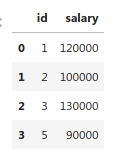As we can see, in this example, each employee has an id associated. We will use this id to merge both DataFrames.

Note that there is an employee named James whose id is 4, but our salaries dataframe doesn’t have an id 4. Similarly, there is a salary with id 5, but we do not have an employee with id 5. We will cover all scenarios, so we have four possibilities for the final merged dataframe:
1- Keeping all records and filling values with NaNs. (Outer Join)
2- Keeping only those records whose id appear on both dataframes. (Inner Join)
3- Keeping only those records with name values and filling missing salaries with NaNs. (Left Join)
4- Keeping only those records with salary values and filling missing names with NaNs. (Right Join)

These are the four types of joins when merging data:
1- Outer
2- Inner
3- Left
4- Right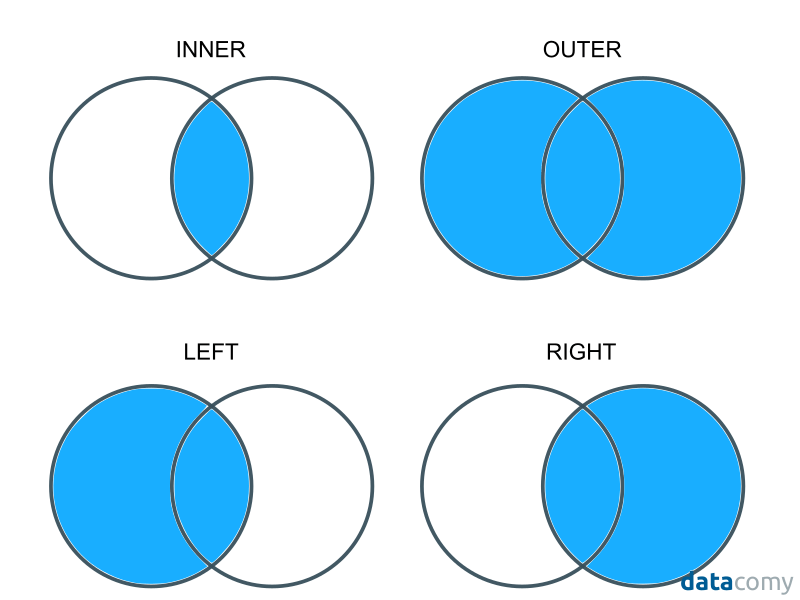import pandas as pd

# Our employees' id and names
employees = pd.DataFrame({'id':[1,2,3,4],'name':['Johana','Mike','Patricia','James']})
employees


id name
0 1 Johana
1 2 Mike
2 3 Patricia
3 4 James
# Our employees' id and salary
salary = pd.DataFrame({'id':[1,2,3,5],'salary':[120000,100000,130000,90000]})
salary


id salary
0 1 120000
1 2 100000
2 3 130000
3 5 90000

## Inner Join

The inner join is the most common type of join and the default for the pandas.DataFrame.merge method

employees_with_salary = employees.merge(salary,
left_on="id",
right_on="id",
how='inner')  # default
employees_with_salary


id name salary
0 1 Johana 120000
1 2 Mike 100000
2 3 Patricia 130000

The type of join is specified with the parameter how. When performing an inner join, as seen above, we don’t need to specify this parameter as it’s the default.

inner uses the intersection of both dataframes. In our case, we can see that:

• James has id 4, but id 4 is not in the salary dataframe.
• From the salary dataframe, there is an employee with id 5, but there is no id 5 in the employees dataframe

The resulting dataset from an inner join doesn’t have James nor employee with id 5. This introduces us to the next type of join: the outer join.

inner is the default value for the how argument, so you can omit it when performing an inner merge, as you can see below:

employees_with_salary2 = employees.merge(salary,
left_on="id",
right_on="id")
employees_with_salary2


id name salary
0 1 Johana 120000
1 2 Mike 100000
2 3 Patricia 130000# Outer Join

The outer join uses the union from both dataframes. Remember that the parameter for specifying the type of join is how.

employees_with_salary_outer = employees.merge(salary,
left_on='id',
right_on='id',
how='outer')
employees_with_salary_outer


id name salary
0 1 Johana 120000.0
1 2 Mike 100000.0
2 3 Patricia 130000.0
3 4 James NaN
4 5 NaN 90000.0

We see that the employee with id 4 is included. Since this name is not in the employees dataframe, a NaN value is instead of his name.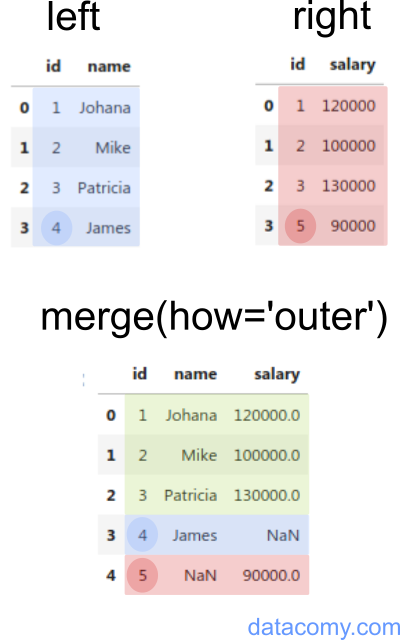## Left Join

You probably already found by yourself how joins are performed when using the how='left' and how='right' parameters.

In any case, the left join uses the keys from the left frame. In our case, James will still be on the merged dataframe:

employees_with_salary_left = employees.merge(salary,
left_on='id',
right_on='id',
how='left')
employees_with_salary_left


id name salary
0 1 Johana 120000.0
1 2 Mike 100000.0
2 3 Patricia 130000.0
3 4 James NaN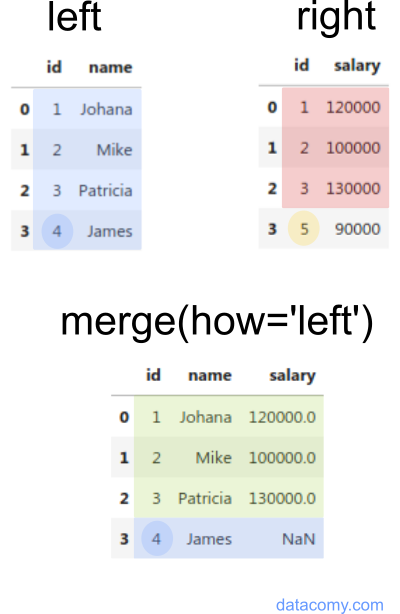## Right Join

And if we use the how='right' parameter, James will be left out (id 4 is not present on the salary dataframe); but the employee whose id is 5 will be in the resulting dataframe.

employees_with_salary_right = employees.merge(salary,
left_on='id',
right_on='id',
how='right')
employees_with_salary_right


id name salary
0 1 Johana 120000
1 2 Mike 100000
2 3 Patricia 130000
3 5 NaN 90000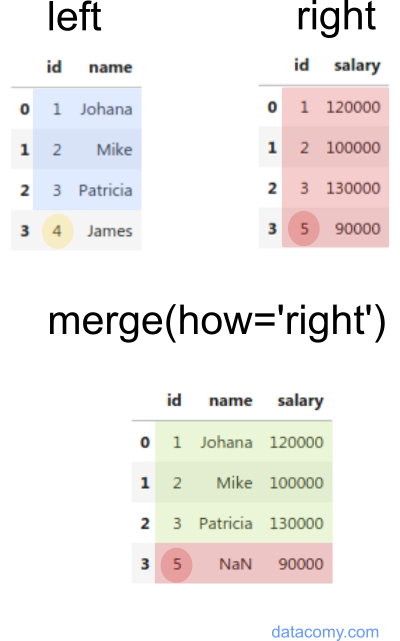## Several ways to specify which columns you want to use to merge the data

You can tell .merge() which columns you want to use to merge the data in several ways:

• on.
• left_on and right_on.
• left_index and right_index.
• Default: can be used when the dataframes share columns with the same name (see below).

## On

The on argument is used to tell merge() which columns you want to use to merge the data.

on can be used only when both Dataframes have a column with keys in common.

In our above example, both Dataframes had a column named id, so we could just use on instead of left_on and right_on:

employees.merge(salary, on="id")  # this is equivalent to left_on="id",right_on="id"


id name salary
0 1 Johana 120000
1 2 Mike 100000
2 3 Patricia 130000

## left_on and right_on

These arguments are used to specify columns or index you want to use as the key for merging the Dataframes. Both default to None.

So, if we wanted to merge 2 Dataframes on different column names, we can use left_on and right_on:

countries = pd.DataFrame({"country": ["United States", "China"], "code": ["US", "CN"]})
countries


country code
0 United States US
1 China CN
population = pd.DataFrame({"country_c": ["CN", "US"], "pop": [1405, 330]})
population


country_c pop
0 CN 1405
1 US 330

In this case, we want to merge on country code, but the columns are named code in the first Dataframe and country_c in the second one. So we must specify the key columns using left_on and right_on:

countries.merge(population, left_on='code', right_on='country_c')


country code country_c pop
0 United States US US 330
1 China CN CN 1405

As we can see, the resulting dataframe has two columns for the country code:
code and country_c. One way to avoid having duplicate data would be to just drop one of the columns:

countries.merge(population, left_on="code", right_on="country_c").drop(
columns=["country_c"]
)


country code pop
0 United States US 330
1 China CN 1405

## What is the difference between pd.merge and df.merge?

df1.merge(df2) and pd.merge(df1, df2) are equivalent, because left_df.merge() calls pd.merge()

Sometimes, df1.merge(df2) is useful because it allows consistent method chaining. For example, if you had to merge 3 DataFrames:

df1 = pd.DataFrame({'letter': ['a', 'b', 'c'], 'a': [1, 2, 3]})
df2 = pd.DataFrame({'letter': ['a', 'b', 'c'], 'b': [4, 5, 6]})
df3 = pd.DataFrame({'letter': ['a', 'b', 'c'], 'c': [7, 8, 9]})
df1.merge(df2).merge(df3)


letter a b c
0 a 1 4 7
1 b 2 5 8
2 c 3 6 9

## Merge on Multiple Columns

If we wanted to join on multiple columns, we can pass a list of columns to the on argument.

df1 = pd.DataFrame({
'letter1': ['a', 'b', 'c'],
'letter2': ['x', 'y', 'z'],
'a': [1, 2, 3]
})
df2 = pd.DataFrame({
'letter1': ['a', 'b', 'c'],
'letter2': ['x', 'y', 'z'],
'b': [4, 5, 6]
})
df1.merge(df2,
left_on=['letter1', 'letter2'],
right_on=['letter1', 'letter2']
)


letter1 letter2 a b
0 a x 1 4
1 b y 2 5
2 c z 3 6

If on is None (the default), pandas will try to merge using common columns found in both dataframes:

df1.merge(df2)


letter1 letter2 a b
0 a x 1 4
1 b y 2 5
2 c z 3 6

## How to Keep index when using Pandas Merge

By default, Pandas merge creates a new integer index for the merged DataFrame.

If we wanted to preserve the index from the first DataFrame as the index of the merged DataFrame, we can specify the index explicitly using .set_axis(df1.index) on the merged DataFrame:

.set_axis() assigns a desired index to given axis. The default value is 0 which means rows.

df1 = pd.DataFrame({
'letter': ['a', 'b', 'c'],
'a': [1, 2, 3]
}, index=['x', 'y', 'z'])
df2 = pd.DataFrame({
'letter': ['a', 'b', 'c'],
'b': [4, 5, 6]
}, index=['xx', 'yy', 'zz'])
df1.merge(df2).set_axis(df1.index)


letter a b
x a 1 4
y b 2 5
z c 3 6
Previous
Next